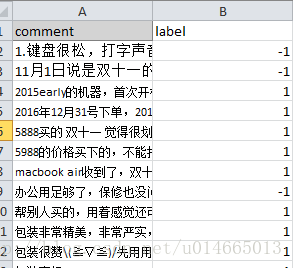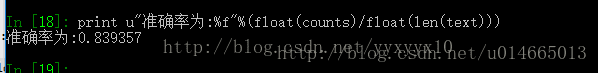• 一、snownlp简介snownlp是什么？SnowNLP是一个python写的类库，可以方便的处理中文文本内容，是受到了TextBlob的启发而写的，由于现在大部分的自然语言处理库基本都是针对英文的，于是写了一个方便处理中文的类库，...
一、snownlp简介snownlp是什么？SnowNLP是一个python写的类库，可以方便的处理中文文本内容，是受到了TextBlob的启发而写的，由于现在大部分的自然语言处理库基本都是针对英文的，于是写了一个方便处理中文的类库，并且和TextBlob不同的是，这里没有用NLTK，所有的算法都是自己实现的，并且自带了一些训练好的字典。注意本程序都是处理的unicode编码，所以使用时请自行decode成unicode。以上是官方对snownlp的描述，简单地说，snownlp是一个中文的自然语言处理的Python库，支持的中文自然语言操作包括：中文分词词性标注情感分析文本分类转换成拼音繁体转简体提取文本关键词提取文本摘要tf，idfTokenization文本相似在本文中，将重点介绍snownlp中的情感分析(Sentiment Analysis)。二、snownlp情感分析模块的使用2.1、snownlp库的安装snownlp的安装方法如下：pip install snownlp2.2、使用snownlp情感分析利用snownlp进行情感分析的代码如下所示：#coding:UTF-8import sysfrom snownlp import SnowNLPdef read_and_analysis(input_file, output_file):f = open(input_file)fw = open(output_file, "w")while True:line = f.readline()if not line:breaklines = line.strip().split("\t")if len(lines) < 2:continues = SnowNLP(lines.decode('utf-8'))# s.words 查询分词结果seg_words = ""for x in s.words:seg_words += "_"seg_words += x# s.sentiments 查询最终的情感分析的得分fw.write(lines + "\t" + lines + "\t" + seg_words.encode('utf-8') + "\t" + str(s.sentiments) + "\n")fw.close()f.close()if __name__ == "__main__":input_file = sys.argvoutput_file = sys.argvread_and_analysis(input_file, output_file)上述代码会从文件中读取每一行的文本，并对其进行情感分析并输出最终的结果。注：库中已经训练好的模型是基于商品的评论数据，因此，在实际使用的过程中，需要根据自己的情况，重新训练模型。2.3、利用新的数据训练情感分析模型在实际的项目中，需要根据实际的数据重新训练情感分析的模型，大致分为如下的几个步骤：准备正负样本，并分别保存，如正样本保存到pos.txt，负样本保存到neg.txt；利用snownlp训练新的模型保存好新的模型重新训练情感分析的代码如下所示：#coding:UTF-8from snownlp import sentimentif __name__ == "__main__":# 重新训练模型sentiment.train('./neg.txt', './pos.txt')# 保存好新训练的模型sentiment.save('sentiment.marshal')注意：若是想要利用新训练的模型进行情感分析，需要修改代码中的调用模型的位置。data_path = os.path.join(os.path.dirname(os.path.abspath(__file__)),'sentiment.marshal')三、snownlp情感分析的源码解析snownlp中支持情感分析的模块在sentiment文件夹中，其核心代码为__init__.py如下是Sentiment类的代码：class Sentiment(object):def __init__(self):self.classifier = Bayes() # 使用的是Bayes的模型def save(self, fname, iszip=True):self.classifier.save(fname, iszip) # 保存最终的模型def load(self, fname=data_path, iszip=True):self.classifier.load(fname, iszip) # 加载贝叶斯模型# 分词以及去停用词的操作def handle(self, doc):words = seg.seg(doc) # 分词words = normal.filter_stop(words) # 去停用词return words # 返回分词后的结果def train(self, neg_docs, pos_docs):data = []# 读入负样本for sent in neg_docs:data.append([self.handle(sent), 'neg'])# 读入正样本for sent in pos_docs:data.append([self.handle(sent), 'pos'])# 调用的是Bayes模型的训练方法self.classifier.train(data)def classify(self, sent):# 1、调用sentiment类中的handle方法# 2、调用Bayes类中的classify方法ret, prob = self.classifier.classify(self.handle(sent)) # 调用贝叶斯中的classify方法if ret == 'pos':return probreturn 1-probclass Sentiment(object):def __init__(self):self.classifier = Bayes() # 使用的是Bayes的模型def save(self, fname, iszip=True):self.classifier.save(fname, iszip) # 保存最终的模型def load(self, fname=data_path, iszip=True):self.classifier.load(fname, iszip) # 加载贝叶斯模型# 分词以及去停用词的操作def handle(self, doc):words = seg.seg(doc) # 分词words = normal.filter_stop(words) # 去停用词return words # 返回分词后的结果def train(self, neg_docs, pos_docs):data = []# 读入负样本for sent in neg_docs:data.append([self.handle(sent), 'neg'])# 读入正样本for sent in pos_docs:data.append([self.handle(sent), 'pos'])# 调用的是Bayes模型的训练方法self.classifier.train(data)def classify(self, sent):# 1、调用sentiment类中的handle方法# 2、调用Bayes类中的classify方法ret, prob = self.classifier.classify(self.handle(sent)) # 调用贝叶斯中的classify方法if ret == 'pos':return probreturn 1-prob从上述的代码中，classify函数和train函数是两个核心的函数，其中，train函数用于训练一个情感分类器，classify函数用于预测。在这两个函数中，都同时使用到的handle函数，handle函数的主要工作为：对输入文本分词去停用词情感分类的基本模型是贝叶斯模型Bayes，对于贝叶斯模型，可以参见文章简单易学的机器学习算法——朴素贝叶斯。对于有两个类别c1c1和c2c2的分类问题来说，其特征为w1,⋯,wnw1,⋯,wn，特征之间是相互独立的，属于类别c1c1的贝叶斯模型的基本过程为：P(c1∣w1,⋯,wn)=P(w1,⋯,wn∣c1)⋅P(c1)P(w1,⋯,wn)P(c1∣w1,⋯,wn)=P(w1,⋯,wn∣c1)⋅P(c1)P(w1,⋯,wn)其中：P(w1,⋯,wn)=P(w1,⋯,wn∣c1)⋅P(c1)+P(w1,⋯,wn∣c2)⋅P(c2)P(w1,⋯,wn)=P(w1,⋯,wn∣c1)⋅P(c1)+P(w1,⋯,wn∣c2)⋅P(c2)3.1、贝叶斯模型的训练贝叶斯模型的训练过程实质上是在统计每一个特征出现的频次，其核心代码如下：def train(self, data):# data 中既包含正样本，也包含负样本for d in data: # data中是list# d:分词的结果，list# d:正/负样本的标记c = dif c not in self.d:self.d[c] = AddOneProb() # 类的初始化for word in d: # 分词结果中的每一个词self.d[c].add(word, 1)# 返回的是正类和负类之和self.total = sum(map(lambda x: self.d[x].getsum(), self.d.keys())) # 取得所有的d中的sum之和这使用到了AddOneProb类，AddOneProb类如下所示：class AddOneProb(BaseProb):def __init__(self):self.d = {}self.total = 0.0self.none = 1 # 默认所有的none为1# 这里如果value也等于1，则当key不存在时，累加的是2def add(self, key, value):self.total += value# 不存在该key时，需新建keyif not self.exists(key):self.d[key] = 1self.total += 1self.d[key] += value注意：none的默认值为1当key不存在时，total和对应的d[key]累加的是1+value，这在后面预测时需要用到AddOneProb类中的total表示的是正类或者负类中的所有值；train函数中的total表示的是正负类的total之和。当统计好了训练样本中的total和每一个特征key的d[key]后，训练过程就构建完成了。3.2、贝叶斯模型的预测预测的过程使用到了上述的公式，即：P(c1∣w1,⋯,wn)=P(w1,⋯,wn∣c1)⋅P(c1)P(w1,⋯,wn∣c1)⋅P(c1)+P(w1,⋯,wn∣c2)⋅P(c2)P(c1∣w1,⋯,wn)=P(w1,⋯,wn∣c1)⋅P(c1)P(w1,⋯,wn∣c1)⋅P(c1)+P(w1,⋯,wn∣c2)⋅P(c2)对上述的公式简化：P(c1∣w1,⋯,wn)=P(w1,⋯,wn∣c1)⋅P(c1)P(w1,⋯,wn∣c1)⋅P(c1)+P(w1,⋯,wn∣c2)⋅P(c2)=11+P(w1,⋯,wn∣c2)⋅P(c2)P(w1,⋯,wn∣c1)⋅P(c1)=11+exp[log(P(w1,⋯,wn∣c2)⋅P(c2)P(w1,⋯,wn∣c1)⋅P(c1))]=11+exp[log(P(w1,⋯,wn∣c2)⋅P(c2))−log(P(w1,⋯,wn∣c1)⋅P(c1))]P(c1∣w1,⋯,wn)=P(w1,⋯,wn∣c1)⋅P(c1)P(w1,⋯,wn∣c1)⋅P(c1)+P(w1,⋯,wn∣c2)⋅P(c2)=11+P(w1,⋯,wn∣c2)⋅P(c2)P(w1,⋯,wn∣c1)⋅P(c1)=11+exp[log(P(w1,⋯,wn∣c2)⋅P(c2)P(w1,⋯,wn∣c1)⋅P(c1))]=11+exp[log(P(w1,⋯,wn∣c2)⋅P(c2))−log(P(w1,⋯,wn∣c1)⋅P(c1))]其中，分母中的1可以改写为：1=exp[log(P(w1,⋯,wn∣c1)⋅P(c1))−log(P(w1,⋯,wn∣c1)⋅P(c1))]1=exp[log(P(w1,⋯,wn∣c1)⋅P(c1))−log(P(w1,⋯,wn∣c1)⋅P(c1))]上述过程对应的代码如下所示：def classify(self, x):tmp = {}for k in self.d: # 正类和负类tmp[k] = log(self.d[k].getsum()) - log(self.total) # 正类/负类的和的log函数-所有之和的log函数for word in x:tmp[k] += log(self.d[k].freq(word)) # 词频，不存在就为0ret, prob = 0, 0for k in self.d:now = 0try:for otherk in self.d:now += exp(tmp[otherk]-tmp[k])now = 1/nowexcept OverflowError:now = 0if now > prob:ret, prob = k, nowreturn (ret, prob)其中，第一个for循环中的tmp[k]对应了公式中的log(P(ck))log(P(ck))，第二个for循环中的tmp[k]对应了公式中的log(P(w1,⋯,wn∣ck)⋅P(ck))log(P(w1,⋯,wn∣ck)⋅P(ck))。参考文献转：https://blog.csdn.net/google19890102/article/details/80091502标签：log,wn,self,实践,snownlp,情感,w1,c2,c1来源： https://www.cnblogs.com/presleyren/p/14210909.html
展开全文• 昨晚上发现了snownlp这个库，很开心。先说说我开心的原因。我本科毕业设计做的是文本挖掘，用R语言做的，发现R语言对文本处理特别不友好，没有很多强大的库，特别是针对中文文本的，加上那时候还没有学机器学习算法...
昨晚上发现了snownlp这个库，很开心。先说说我开心的原因。我本科毕业设计做的是文本挖掘，用R语言做的，发现R语言对文本处理特别不友好，没有很多强大的库，特别是针对中文文本的，加上那时候还没有学机器学习算法。所以很头疼，后来不得已用了一个可视化的软件RostCM，但是一般可视化软件最大的缺点是无法调参，很死板，准确率并不高。现在研一，机器学习算法学完以后，又想起来要继续学习文本挖掘了。所以前半个月开始了用python进行文本挖掘的学习，很多人都推荐我从《python自然语言处理》这本书入门，学习了半个月以后，可能本科毕业设计的时候有些基础了，再看这个感觉没太多进步，并且这里通篇将nltk库进行英文文本挖掘的，英文文本挖掘跟中文是有很大差别的，或者说学完英文文本挖掘，再做中文的，也是完全懵逼的。所以我停了下来，觉得太没效率了。然后我在网上查找关于python如何进行中文文本挖掘的文章，最后找到了snownlp这个库，这个库是国人自己开发的python类库，专门针对中文文本进行挖掘，里面已经有了算法，需要自己调用函数，根据不同的文本构建语料库就可以，真的太方便了。我只介绍一下这个库具体应用，不介绍其中的有关算法原理，因为算法原理可以自己去学习。因为我在学习这个库的时候，我查了很多资料发现很少或者基本没有写这个库的实例应用，很多都是转载官网对这个库的简介，所以我记录一下我今天的学习。首先简单介绍一下这个库可以进行哪些文本挖掘。snownlp主要可以进行中文分词(算法是Character-Based Generative Model)、词性标注(原理是TnT、3-gram 隐马)、情感分析(官网木有介绍原理，但是指明购物类的评论的准确率较高，其实是因为它的语料库主要是购物方面的，可以自己构建相关领域语料库，替换原来的，准确率也挺不错的)、文本分类(原理是朴素贝叶斯)、转换拼音、繁体转简体、提取文本关键词(原理是TextRank)、提取摘要(原理是TextRank)、分割句子、文本相似(原理是BM25)。官网还有更多关于该库的介绍，在看我这个文章之前，建议先看一下官网，里面有最基础的一些命令的介绍。官网链接：https://pypi.python.org/pypi/snownlp/0.11.1。下面正式介绍实例应用。主要是中文文本的情感分析，我今天从京东网站采集了249条关于笔记本的评论文本作为练习数据，由于我只是想练习一下，没采集更多。然后人工标注每条评论的情感正负性，情感正负性就是指该条评论代表了评论者的何种态度，是褒义还是贬义。以下是样例：其中-1表示贬义，1表示褒义。由于snownlp全部是unicode编码，所以要注意数据是否为unicode编码。因为是unicode编码，所以不需要去除中文文本里面含有的英文，因为都会被转码成统一的编码(补充一下，关于编码问题，我还是不特别清楚，所以这里不多讲，还请对这方面比较熟悉的伙伴多多指教)。软件本身默认的是Ascii编码，所以第一步先设置软件的默认编码为utf-8，代码如下：1、改变软件默认编码import sysreload(sys)sys.setdefaultencoding('utf-8')2、然后准备数据import pandas as pd #加载pandastext=pd.read_excel(u'F:/自然语言处理/评论文本.xlsx',header=0) #读取文本数据text0=text.iloc[:,0] #提取所有数据text1=[i.decode('utf-8') for i in text0] #上一步提取数据不是字符而是object，所以在这一步进行转码为字符3、训练语料库from snownlp import sentiment #加载情感分析模块sentiment.train('E:/Anaconda2/Lib/site-packages/snownlp/sentiment/neg.txt', 'E:/Anaconda2/Lib/site-packages/snownlp/sentiment/pos.txt') #对语料库进行训练，把路径改成相应的位置。我这次练习并没有构建语料库，用了默认的，所以把路径写到了sentiment模块下。sentiment.save('D:/pyscript/sentiment.marshal')#这一步是对上一步的训练结果进行保存，如果以后语料库没有改变，下次不用再进行训练，直接使用就可以了，所以一定要保存，保存位置可以自己决定，但是要把snownlp/seg/__init__.py里的data_path也改成你保存的位置，不然下次使用还是默认的。4、进行预测from snownlp import SnowNLPsenti=[SnowNLP(i).sentiments for i in text1] #遍历每条评论进行预测5、进行验证准确率预测结果为positive的概率，positive的概率大于等于0.6，我认为可以判断为积极情感，小于0.6的判断为消极情感。所以以下将概率大于等于0.6的评论标签赋为1，小于0.6的评论标签赋为-1，方便后面与实际标签进行比较。newsenti=[]for i in senti:if (i>=0.6):newsenti.append(1)else:newsenti.append(-1)text['predict']=newsenti  #将新的预测标签增加为text的某一列，所以现在text的第0列为评论文本，第1列为实际标签，第2列为预测标签counts=0for j in range(len(text.iloc[:,0])): #遍历所有标签，将预测标签和实际标签进行比较，相同则判断正确。if text.iloc[j,2]==text.iloc[j,1]:counts+=1print u"准确率为:%f"%(float(counts)/float(len(text)))#输出本次预测的准确率运行结果为：准确率还可以，但还不算高，原因是我考虑时间原因，并且我只是练习一下，所以没有自己构建该领域的语料库，如果构建了相关语料库，替换默认语料库，准确率会高很多。所以语料库是非常关键的，如果要正式进行文本挖掘，建议要构建自己的语料库。在没有构建新的语料库的情况下，这个83.9357%的准确率还是不错了。以上是我这次的学习笔记，和大家分享一下，有不足之处请大家批评指正。我还是一个刚涉世数据挖掘、机器学习、文本挖掘领域不久的小白，有许多知识还是比较模糊，但对这数据挖掘很感兴趣。希望能多结识这方面的朋友，共同学习、共同进步。转自：博客
展开全文• snownlp主要可以进行中文分词(算法是Character-Based Generative Model)、词性标注(原理是TnT、3-gram 隐马)、情感分析(官网木有介绍原理，但是指明购物类的评论的准确率较高，其实是因为它的语料库主要是购物方面的...
昨晚上发现了snownlp这个库，很开心。首先简单介绍一下这个库可以进行哪些文本挖掘。snownlp主要可以进行中文分词(算法是Character-Based Generative Model)、词性标注(原理是TnT、3-gram 隐马)、情感分析(官网木有介绍原理，但是指明购物类的评论的准确率较高，其实是因为它的语料库主要是购物方面的，可以自己构建相关领域语料库，替换原来的，准确率也挺不错的)、文本分类(原理是朴素贝叶斯)、转换拼音、繁体转简体、提取文本关键词(原理是TextRank)、提取摘要(原理是TextRank)、分割句子、文本相似(原理是BM25)。官网还有更多关于该库的介绍，在看我这个文章之前，建议先看一下官网，里面有最基础的一些命令的介绍。官网链接：https://pypi.python.org/pypi/snownlp/0.11.1。下面正式介绍实例应用。主要是中文文本的情感分析，我今天从京东网站采集了249条关于笔记本的评论文本作为练习数据，由于我只是想练习一下，没采集更多。然后人工标注每条评论的情感正负性，情感正负性就是指该条评论代表了评论者的何种态度，是褒义还是贬义。以下是样例其中-1表示贬义，1表示褒义。由于snownlp全部是unicode编码，所以要注意数据是否为unicode编码。因为是unicode编码，所以不需要去除中文文本里面含有的英文，因为都会被转码成统一的编码(补充一下，关于编码问题，我还是不特别清楚，所以这里不多讲，还请对这方面比较熟悉的伙伴多多指教)。软件本身默认的是Ascii编码，所以第一步先设置软件的默认编码为utf-8，代码如下：1、改变软件默认编码import sysreload(sys)sys.setdefaultencoding('utf-8')2、然后准备数据import pandas as pd #加载pandastext=pd.read_excel(u'F:/自然语言处理/评论文本.xlsx',header=0) #读取文本数据text0=text.iloc[:,0] #提取所有数据text1=[i.decode('utf-8') for i in text0] #上一步提取数据不是字符而是object，所以在这一步进行转码为字符3、训练语料库from snownlp import sentiment #加载情感分析模块sentiment.train('E:/Anaconda2/Lib/site-packages/snownlp/sentiment/neg.txt', 'E:/Anaconda2/Lib/site-packages/snownlp/sentiment/pos.txt') #对语料库进行训练，把路径改成相应的位置。我这次练习并没有构建语料库，用了默认的，所以把路径写到了sentiment模块下。sentiment.save('D:/pyscript/sentiment.marshal')#这一步是对上一步的训练结果进行保存，如果以后语料库没有改变，下次不用再进行训练，直接使用就可以了，所以一定要保存，保存位置可以自己决定，但是要把snownlp/seg/__init__.py里的data_path也改成你保存的位置，不然下次使用还是默认的。4、进行预测from snownlp import SnowNLPsenti=[SnowNLP(i).sentiments for i in text1] #遍历每条评论进行预测5、进行验证准确率预测结果为positive的概率，positive的概率大于等于0.6，我认为可以判断为积极情感，小于0.6的判断为消极情感。所以以下将概率大于等于0.6的评论标签赋为1，小于0.6的评论标签赋为-1，方便后面与实际标签进行比较。newsenti=[]for i in senti:if (i>=0.6):newsenti.append(1)else:newsenti.append(-1)text['predict']=newsenti  #将新的预测标签增加为text的某一列，所以现在text的第0列为评论文本，第1列为实际标签，第2列为预测标签counts=0for j in range(len(text.iloc[:,0])): #遍历所有标签，将预测标签和实际标签进行比较，相同则判断正确。if text.iloc[j,2]==text.iloc[j,1]:counts+=1print u"准确率为:%f"%(float(counts)/float(len(text)))#输出本次预测的准确率运行结果为：准确率还可以，但还不算高，原因是我考虑时间原因，并且我只是练习一下，所以没有自己构建该领域的语料库，如果构建了相关语料库，替换默认语料库，准确率会高很多。所以语料库是非常关键的，如果要正式进行文本挖掘，建议要构建自己的语料库。在没有构建新的语料库的情况下，这个83.9357%的准确率还是不错了。以上是我这次的学习笔记，和大家分享一下，有不足之处请大家批评指正。我还是一个刚涉世数据挖掘、机器学习、文本挖掘领域不久的小白，有许多知识还是比较模糊，但对这数据挖掘很感兴趣。希望能多结识这方面的朋友，共同学习、共同进步。
展开全文• 一、snownlp简介 snownlp是什么？ SnowNLP是一个python写的类库，可以方便的处理中文文本内容，是受到了TextBlob的启发而写的，由于现在大部分的自然语言处理库基本都是针对英文的，于是写了一个方便处理中文的...
一、snownlp简介

snownlp是什么？

SnowNLP是一个python写的类库，可以方便的处理中文文本内容，是受到了TextBlob的启发而写的，由于现在大部分的自然语言处理库基本都是针对英文的，于是写了一个方便处理中文的类库，并且和TextBlob不同的是，这里没有用NLTK，所有的算法都是自己实现的，并且自带了一些训练好的字典。注意本程序都是处理的unicode编码，所以使用时请自行decode成unicode。

以上是官方对snownlp的描述，简单地说，snownlp是一个中文的自然语言处理的Python库，支持的中文自然语言操作包括：

中文分词
词性标注
情感分析
文本分类
转换成拼音
繁体转简体
提取文本关键词
提取文本摘要
tf，idf
Tokenization
文本相似

在本文中，将重点介绍snownlp中的情感分析（Sentiment Analysis）。

二、snownlp情感分析模块的使用

2.1、snownlp库的安装

snownlp的安装方法如下：

pip install snownlp

2.2、使用snownlp情感分析

利用snownlp进行情感分析的代码如下所示：

#coding:UTF-8
import sys
from snownlp import SnowNLP

def read_and_analysis(input_file, output_file):
f = open(input_file)
fw = open(output_file, "w")
while True:
line = f.readline()
if not line:
break
lines = line.strip().split("\t")
if len(lines) < 2:
continue

s = SnowNLP(lines.decode('utf-8'))
# s.words 查询分词结果
seg_words = ""
for x in s.words:
seg_words += "_"
seg_words += x
# s.sentiments 查询最终的情感分析的得分
fw.write(lines + "\t" + lines + "\t" + seg_words.encode('utf-8') + "\t" + str(s.sentiments) + "\n")
fw.close()
f.close()

if __name__ == "__main__":
input_file = sys.argv
output_file = sys.argv
read_and_analysis(input_file, output_file)

上述代码会从文件中读取每一行的文本，并对其进行情感分析并输出最终的结果。

注：库中已经训练好的模型是基于商品的评论数据，因此，在实际使用的过程中，需要根据自己的情况，重新训练模型。

2.3、利用新的数据训练情感分析模型

在实际的项目中，需要根据实际的数据重新训练情感分析的模型，大致分为如下的几个步骤：

准备正负样本，并分别保存，如正样本保存到pos.txt，负样本保存到neg.txt；
利用snownlp训练新的模型
保存好新的模型

重新训练情感分析的代码如下所示：

#coding:UTF-8

from snownlp import sentiment

if __name__ == "__main__":
# 重新训练模型
sentiment.train('./neg.txt', './pos.txt')
# 保存好新训练的模型
sentiment.save('sentiment.marshal')

注意：若是想要利用新训练的模型进行情感分析，需要修改代码中的调用模型的位置。

data_path = os.path.join(os.path.dirname(os.path.abspath(__file__)),'sentiment.marshal')

三、snownlp情感分析的源码解析

snownlp中支持情感分析的模块在sentiment文件夹中，其核心代码为__init__.py

如下是Sentiment类的代码：

class Sentiment(object):

def __init__(self):
self.classifier = Bayes() # 使用的是Bayes的模型

def save(self, fname, iszip=True):
self.classifier.save(fname, iszip) # 保存最终的模型

def load(self, fname=data_path, iszip=True):
self.classifier.load(fname, iszip) # 加载贝叶斯模型

# 分词以及去停用词的操作
def handle(self, doc):
words = seg.seg(doc) # 分词
words = normal.filter_stop(words) # 去停用词
return words # 返回分词后的结果

def train(self, neg_docs, pos_docs):
data = []
# 读入负样本
for sent in neg_docs:
data.append([self.handle(sent), 'neg'])
# 读入正样本
for sent in pos_docs:
data.append([self.handle(sent), 'pos'])
# 调用的是Bayes模型的训练方法
self.classifier.train(data)

def classify(self, sent):
# 1、调用sentiment类中的handle方法
# 2、调用Bayes类中的classify方法
ret, prob = self.classifier.classify(self.handle(sent)) # 调用贝叶斯中的classify方法
if ret == 'pos':
return prob
return 1-probclass Sentiment(object):

def __init__(self):
self.classifier = Bayes() # 使用的是Bayes的模型

def save(self, fname, iszip=True):
self.classifier.save(fname, iszip) # 保存最终的模型

def load(self, fname=data_path, iszip=True):
self.classifier.load(fname, iszip) # 加载贝叶斯模型

# 分词以及去停用词的操作
def handle(self, doc):
words = seg.seg(doc) # 分词
words = normal.filter_stop(words) # 去停用词
return words # 返回分词后的结果

def train(self, neg_docs, pos_docs):
data = []
# 读入负样本
for sent in neg_docs:
data.append([self.handle(sent), 'neg'])
# 读入正样本
for sent in pos_docs:
data.append([self.handle(sent), 'pos'])
# 调用的是Bayes模型的训练方法
self.classifier.train(data)

def classify(self, sent):
# 1、调用sentiment类中的handle方法
# 2、调用Bayes类中的classify方法
ret, prob = self.classifier.classify(self.handle(sent)) # 调用贝叶斯中的classify方法
if ret == 'pos':
return prob
return 1-prob

从上述的代码中，classify函数和train函数是两个核心的函数，其中，train函数用于训练一个情感分类器，classify函数用于预测。在这两个函数中，都同时使用到的handle函数，handle函数的主要工作为：

对输入文本分词
去停用词

情感分类的基本模型是贝叶斯模型Bayes，对于贝叶斯模型，可以参见文章简单易学的机器学习算法——朴素贝叶斯。对于有两个类别c1${c}_{1}$$c_1$和c2${c}_{2}$$c_2$的分类问题来说，其特征为w1,⋯,wn${w}_{1},\cdots ,{w}_{n}$$w_1,\cdots ,w_n$，特征之间是相互独立的，属于类别c1${c}_{1}$$c_1$的贝叶斯模型的基本过程为：

P(c1∣w1,⋯,wn)=P(w1,⋯,wn∣c1)⋅P(c1)P(w1,⋯,wn)$P\left({c}_{1}\mid {w}_{1},\cdots ,{w}_{n}\right)=\frac{P\left({w}_{1},\cdots ,{w}_{n}\mid {c}_{1}\right)\cdot P\left({c}_{1}\right)}{P\left({w}_{1},\cdots ,{w}_{n}\right)}$P\left ( c_1\mid w_1,\cdots ,w_n \right )=\frac{P\left ( w_1,\cdots , w_n\mid c_1 \right )\cdot P(c_1)}{P\left ( w_1,\cdots ,w_n \right )}

其中：

P(w1,⋯,wn)=P(w1,⋯,wn∣c1)⋅P(c1)+P(w1,⋯,wn∣c2)⋅P(c2)$P\left({w}_{1},\cdots ,{w}_{n}\right)=P\left({w}_{1},\cdots ,{w}_{n}\mid {c}_{1}\right)\cdot P\left({c}_{1}\right)+P\left({w}_{1},\cdots ,{w}_{n}\mid {c}_{2}\right)\cdot P\left({c}_{2}\right)$P\left ( w_1,\cdots ,w_n \right )=P\left ( w_1,\cdots ,w_n\mid c_1 \right )\cdot P\left ( c_1 \right )+P\left ( w_1,\cdots ,w_n\mid c_2\right )\cdot P\left ( c_2\right )

3.1、贝叶斯模型的训练

贝叶斯模型的训练过程实质上是在统计每一个特征出现的频次，其核心代码如下：

def train(self, data):
# data 中既包含正样本，也包含负样本
for d in data: # data中是list
# d:分词的结果，list
# d:正/负样本的标记
c = d
if c not in self.d:
self.d[c] = AddOneProb() # 类的初始化
for word in d: # 分词结果中的每一个词
self.d[c].add(word, 1)
# 返回的是正类和负类之和
self.total = sum(map(lambda x: self.d[x].getsum(), self.d.keys())) # 取得所有的d中的sum之和

这使用到了AddOneProb类，AddOneProb类如下所示：

class AddOneProb(BaseProb):

def __init__(self):
self.d = {}
self.total = 0.0
self.none = 1 # 默认所有的none为1
# 这里如果value也等于1，则当key不存在时，累加的是2
def add(self, key, value):
self.total += value
# 不存在该key时，需新建key
if not self.exists(key):
self.d[key] = 1
self.total += 1
self.d[key] += value

注意：

none的默认值为1
当key不存在时，total和对应的d[key]累加的是1+value，这在后面预测时需要用到

AddOneProb类中的total表示的是正类或者负类中的所有值；train函数中的total表示的是正负类的total之和。

当统计好了训练样本中的total和每一个特征key的d[key]后，训练过程就构建完成了。

3.2、贝叶斯模型的预测

预测的过程使用到了上述的公式，即：

P(c1∣w1,⋯,wn)=P(w1,⋯,wn∣c1)⋅P(c1)P(w1,⋯,wn∣c1)⋅P(c1)+P(w1,⋯,wn∣c2)⋅P(c2)$P\left({c}_{1}\mid {w}_{1},\cdots ,{w}_{n}\right)=\frac{P\left({w}_{1},\cdots ,{w}_{n}\mid {c}_{1}\right)\cdot P\left({c}_{1}\right)}{P\left({w}_{1},\cdots ,{w}_{n}\mid {c}_{1}\right)\cdot P\left({c}_{1}\right)+P\left({w}_{1},\cdots ,{w}_{n}\mid {c}_{2}\right)\cdot P\left({c}_{2}\right)}$P\left ( c_1\mid w_1,\cdots ,w_n \right )=\frac{P\left ( w_1,\cdots , w_n\mid c_1 \right )\cdot P(c_1)}{P\left ( w_1,\cdots ,w_n\mid c_1 \right )\cdot P\left ( c_1 \right )+P\left ( w_1,\cdots ,w_n\mid c_2\right )\cdot P\left ( c_2\right )}

对上述的公式简化：

P(c1∣w1,⋯,wn)=P(w1,⋯,wn∣c1)⋅P(c1)P(w1,⋯,wn∣c1)⋅P(c1)+P(w1,⋯,wn∣c2)⋅P(c2)=11+P(w1,⋯,wn∣c2)⋅P(c2)P(w1,⋯,wn∣c1)⋅P(c1)=11+exp[log(P(w1,⋯,wn∣c2)⋅P(c2)P(w1,⋯,wn∣c1)⋅P(c1))]=11+exp[log(P(w1,⋯,wn∣c2)⋅P(c2))−log(P(w1,⋯,wn∣c1)⋅P(c1))]$\begin{array}{rl}P\left({c}_{1}\mid {w}_{1},\cdots ,{w}_{n}\right)& =\frac{P\left({w}_{1},\cdots ,{w}_{n}\mid {c}_{1}\right)\cdot P\left({c}_{1}\right)}{P\left({w}_{1},\cdots ,{w}_{n}\mid {c}_{1}\right)\cdot P\left({c}_{1}\right)+P\left({w}_{1},\cdots ,{w}_{n}\mid {c}_{2}\right)\cdot P\left({c}_{2}\right)}\\ & =\frac{1}{1+\frac{P\left({w}_{1},\cdots ,{w}_{n}\mid {c}_{2}\right)\cdot P\left({c}_{2}\right)}{P\left({w}_{1},\cdots ,{w}_{n}\mid {c}_{1}\right)\cdot P\left({c}_{1}\right)}}\\ & =\frac{1}{1+exp\left[log\left(\frac{P\left({w}_{1},\cdots ,{w}_{n}\mid {c}_{2}\right)\cdot P\left({c}_{2}\right)}{P\left({w}_{1},\cdots ,{w}_{n}\mid {c}_{1}\right)\cdot P\left({c}_{1}\right)}\right)\right]}\\ & =\frac{1}{1+exp\left[log\left(P\left({w}_{1},\cdots ,{w}_{n}\mid {c}_{2}\right)\cdot P\left({c}_{2}\right)\right)-log\left(P\left({w}_{1},\cdots ,{w}_{n}\mid {c}_{1}\right)\cdot P\left({c}_{1}\right)\right)\right]}\end{array}$\begin{align*}
P\left ( c_1\mid w_1,\cdots ,w_n \right ) &= \frac{P\left ( w_1,\cdots , w_n\mid c_1 \right )\cdot P(c_1)}{P\left ( w_1,\cdots ,w_n\mid c_1 \right )\cdot P\left ( c_1 \right )+P\left ( w_1,\cdots ,w_n\mid c_2\right )\cdot P\left ( c_2\right )}\\
&= \frac{1}{1+\frac{P\left ( w_1,\cdots ,w_n\mid c_2\right )\cdot P\left ( c_2\right )}{P\left ( w_1,\cdots ,w_n\mid c_1\right )\cdot P\left ( c_1\right )}}\\
&= \frac{1}{1+exp\left [ log\left ( \frac{P\left ( w_1,\cdots ,w_n\mid c_2\right )\cdot P\left ( c_2\right )}{P\left ( w_1,\cdots ,w_n\mid c_1\right )\cdot P\left ( c_1\right )} \right ) \right ]}\\
&= \frac{1}{1+exp\left [ log\left ( P\left ( w_1,\cdots ,w_n\mid c_2\right )\cdot P\left ( c_2\right ) \right )-log\left ( P\left ( w_1,\cdots ,w_n\mid c_1\right )\cdot P\left ( c_1\right ) \right ) \right ]}
\end{align*}

其中，分母中的1可以改写为：

1=exp[log(P(w1,⋯,wn∣c1)⋅P(c1))−log(P(w1,⋯,wn∣c1)⋅P(c1))]$1=exp\left[log\left(P\left({w}_{1},\cdots ,{w}_{n}\mid {c}_{1}\right)\cdot P\left({c}_{1}\right)\right)-log\left(P\left({w}_{1},\cdots ,{w}_{n}\mid {c}_{1}\right)\cdot P\left({c}_{1}\right)\right)\right]$1=exp\left [ log\left ( P\left ( w_1,\cdots ,w_n\mid c_1\right )\cdot P\left ( c_1\right ) \right )-log\left ( P\left ( w_1,\cdots ,w_n\mid c_1\right )\cdot P\left ( c_1\right ) \right ) \right ]

上述过程对应的代码如下所示：

def classify(self, x):
tmp = {}
for k in self.d: # 正类和负类
tmp[k] = log(self.d[k].getsum()) - log(self.total) # 正类/负类的和的log函数-所有之和的log函数
for word in x:
tmp[k] += log(self.d[k].freq(word)) # 词频，不存在就为0
ret, prob = 0, 0
for k in self.d:
now = 0
try:
for otherk in self.d:
now += exp(tmp[otherk]-tmp[k])
now = 1/now
except OverflowError:
now = 0
if now > prob:
ret, prob = k, now
return (ret, prob)

其中，第一个for循环中的tmp[k]对应了公式中的log(P(ck))$log\left(P\left({c}_{k}\right)\right)$$log\left ( P\left ( c_k\right ) \right )$，第二个for循环中的tmp[k]对应了公式中的log(P(w1,⋯,wn∣ck)⋅P(ck))$log\left(P\left({w}_{1},\cdots ,{w}_{n}\mid {c}_{k}\right)\cdot P\left({c}_{k}\right)\right)$$log\left ( P\left ( w_1,\cdots ,w_n\mid c_k\right )\cdot P\left ( c_k\right ) \right )$。

参考文献

snownlp github
自然语言处理库之snowNLP

展开全文情感分析
• snownlps是用Python写的个中文情感分析的包，自带了中文正负情感的训练集，主要是评论的语料库。使用的是朴素贝叶斯原理来训练和预测数据。主要看了一下这个包的几个主要的核心代码，看的过程作了一些注释，记录一下...
• 本文简绍了SnowNLP的使用方法，这是一个处理中文文本内容的python类库，其主要功能包括分词、词性标注、情感分析、汉字转拼音、繁体转简体、关键词提取以及文本摘要等等。简介SnowNLP是一个可以方便的处理中文文本...
• 一、snownlp简介 snownlp是什么？ SnowNLP是一个python写的类库，可以方便的处理中文文本内容，是受到了TextBlob的启发而写的，由于现在大部分的自然语言处理库基本都是针对英文的，于是写了一个方便处理中文的类库...算法 python 机器学习 深度学习 java
• 朋友给我介绍了情感分析的一个RNN算法的，考虑了句子的结构，原理上我懂，但是RNN谁懂啊，神经网络向来复杂，而且它那个的训练集也是22w的正向，20w的负能量，还带上了人工挑选的词汇分值表，内存消耗想必也挺可观的...
• 《老钱------AI实战训练营的系列课程》= 多个案例学习《词性标注》 文本情感分析一直是NLP领域的热门应用，常用在舆情分析，...使用thulac进行词性标注 8.Thulac与Snownlp与结巴分词进行对比 9.名词应用 10.项目总结
• 聊一聊snownlp源代码中的朴素贝叶斯分类原理一、情感分析任务二、分类原理三、snownlp源码解析四、致谢： 建议拿出小本本，写写画画便于理解 一、情感分析任务 简单来说，给一句话，运用情感分析，判断这句话是...NLP
• snownlp主要可以进行中文分词（算法是Character-Based Generative Model）、词性标注（原理是TnT、3-gram 隐马）、情感分析（官网木有介绍原理，但是指明购物类的评论的准确率较高，其实是因为它的语料库主要是购物...机器学习
• snownlps是用Python写的个中文情感分析的包，自带了中文正负情感的训练集，主要是评论的语料库。使用的是朴素贝叶斯原理来训练和预测数据。主要看了一下这个包的几个主要的核心代码，看的过程作了一些注释，记录一下...
• snownlp主要可以进行中文分词（算法是Character-Based Generative Model）、词性标注（原理是TnT、3-gram 隐马）、情感分析（官网木有介绍原理，但是指明购物类的评论的准确率较高，其实是因为它的语料库主要是购物...python
• 现在有很多可以使用的第三方工具包可以很容易的来实现这个功能，比如snowNLP，jieba等，但是我们还是要做到知其然，知其所以然，所以便有了这一篇文字的记录。 首先我们来了解一下什么是TF-IDF？ 其实这个是两个...
• ## 中文分词原理及分词工具介绍

万次阅读 多人点赞 2018-07-31 11:00:37
本文首先介绍下中文分词的基本原理，然后介绍下国内比较流行的中文分词工具，如jieba、SnowNLP、THULAC、NLPIR，上述分词工具都已经在github上开源，后续也会附上github链接，以供参考。 1.中文分词原理介绍 1.1 ...
• 本文首先介绍下中文分词的基本原理，然后介绍下国内比较流行的中文分词工具，如jieba、SnowNLP、THULAC、NLPIR，上述分词工具都已经在github上开源，后续也会附上github链接，以供参考。 1.中文分词原理介绍 1.1 ...分词 jieba python 自然语言处理
• 本文首先介绍下中文分词的基本原理，然后介绍下国内比较流行的中文分词工具，如jieba、SnowNLP、THULAC、NLPIR，上述分词工具都已经在github上开源，后续也会附上github链接，以供参考。 1.中文分词原理介绍 1.1 ...自然语言处理 NLP 中文分词工具 python
• 本文首先介绍下中文分词的基本原理，然后介绍下国内比较流行的中文分词工具，如jieba、SnowNLP、THULAC、NLPIR，上述分词工具都已经在github上开源，后续也会附上github链接，以供参考。 1.中文分词原理介绍 1.1 ...自然语言处理
• 本文首先介绍下中文分词的基本原理，然后介绍下国内比较流行的中文分词工具，如jieba、SnowNLP、THULAC、NLPIR，上述分词工具都已经在github上开源，后续也会附上github链接，以供参考。 1.中文分词原理介绍 1.1 ...